# Part A As a technician in a large pharmaceutical research firm, you need to produce 350....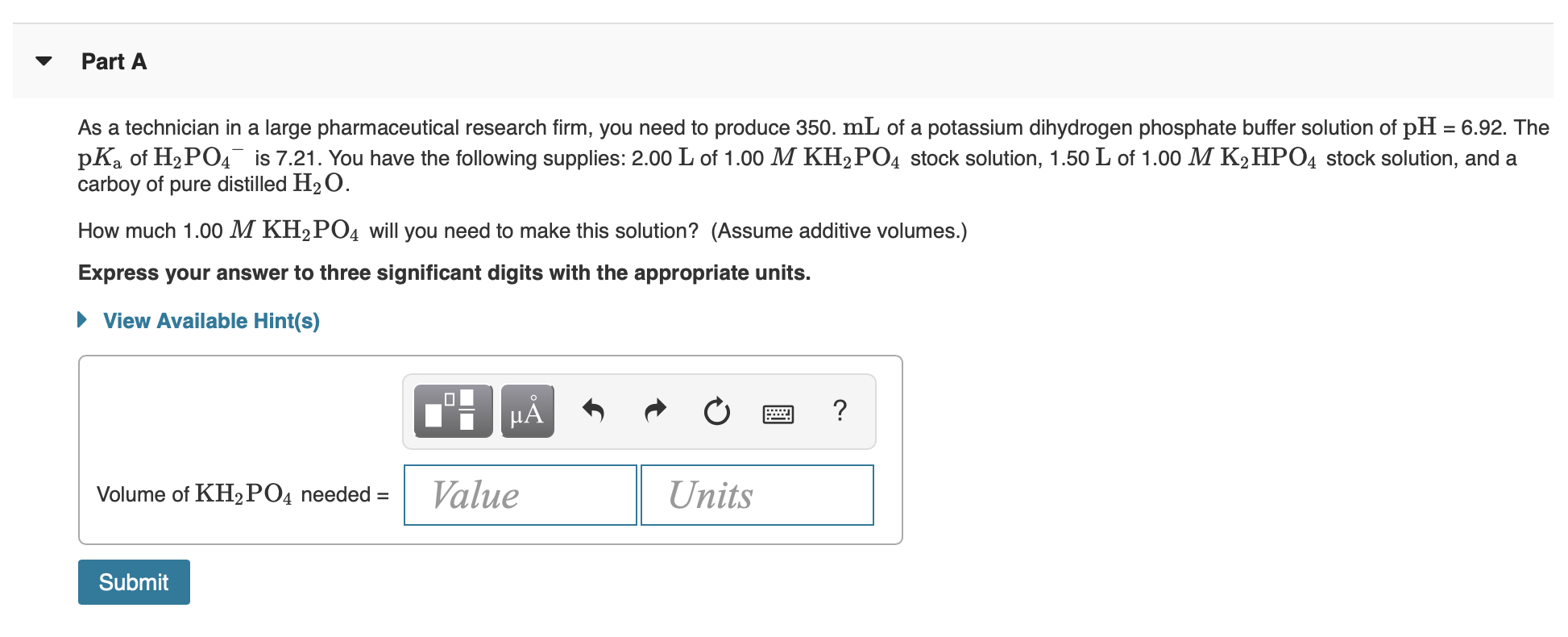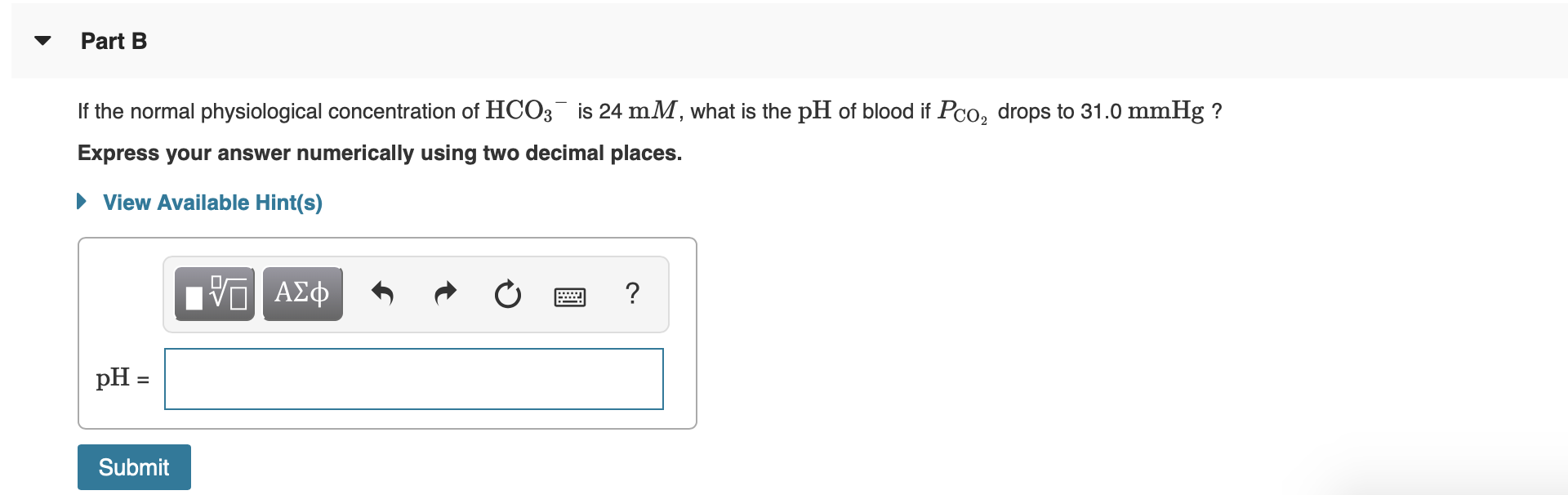Part A As a technician in a large pharmaceutical research firm, you need to produce 350. mL of a potassium dihydrogen phosphate buffer solution of pH = 6.92. The pKa of H2PO4 is 7.21. You have the following supplies: 2.00 L of 1.00 M KH2PO4 stock solution, 1.50 L of 1.00 M K2HPO4 stock solution, and a carboy of pure distilled H2O. How much 1.00 M KH2PO4 will you need to make this solution? (Assume additive volumes.) Express your answer to three significant digits with the appropriate units. ► View Available Hint(s) .: HÅR O 2 ? Volume of KH2PO4 needed = Value Units Submit
Part B If the normal physiological concentration of HCO3 is 24 mM, what is the pH of blood if Pco, drops to 31.0 mmHg ? Express your answer numerically using two decimal places. View Available Hint(s) TO ADD A O O ? pH = Submit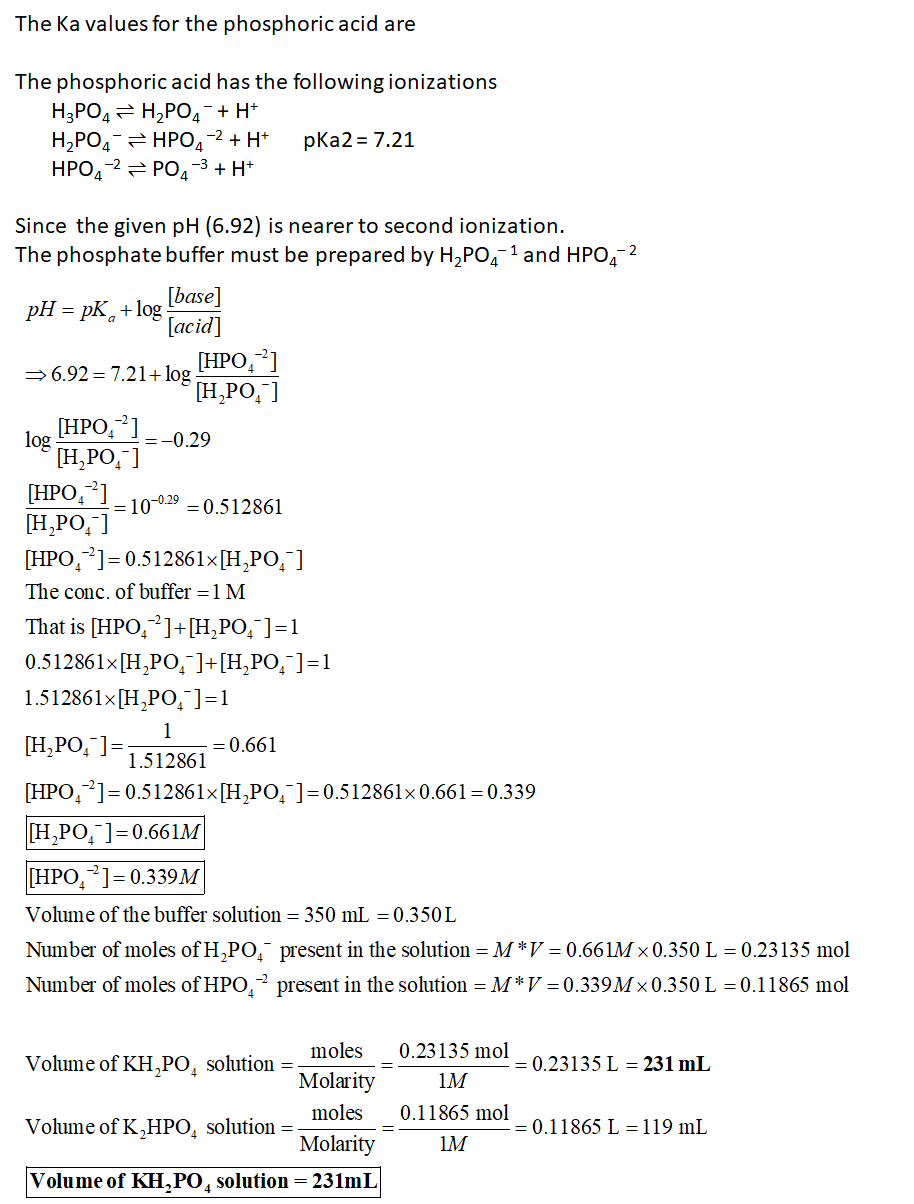============================================================================================================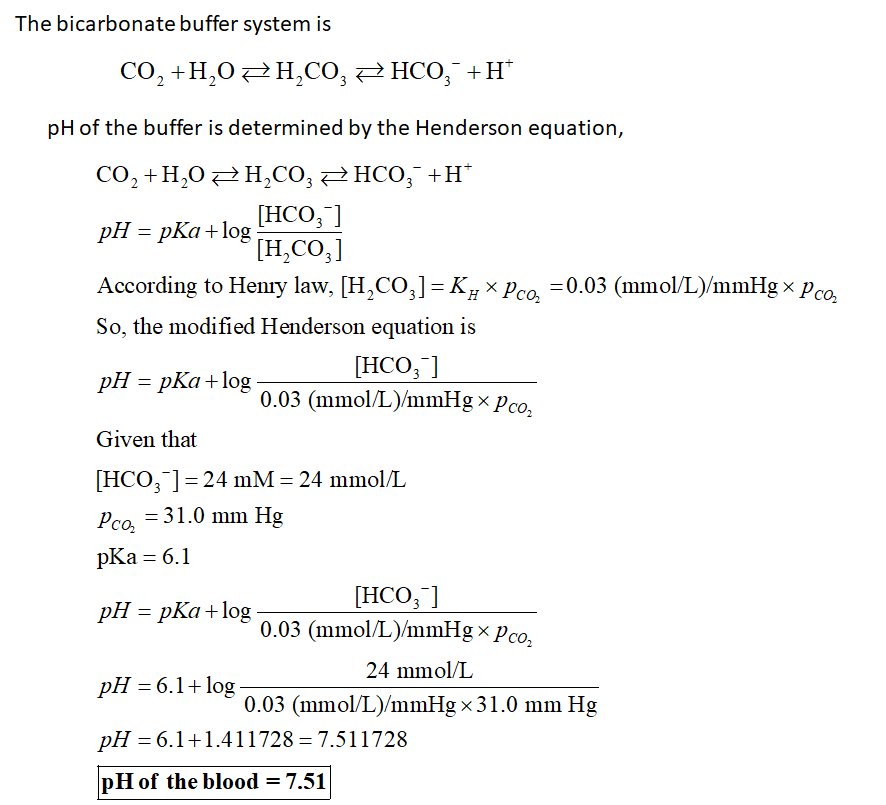#### Earn Coin

Coins can be redeemed for fabulous gifts.

Similar Homework Help Questions
• ### Part A As a technician in a large pharmaceutical research firm, you need to produce 300...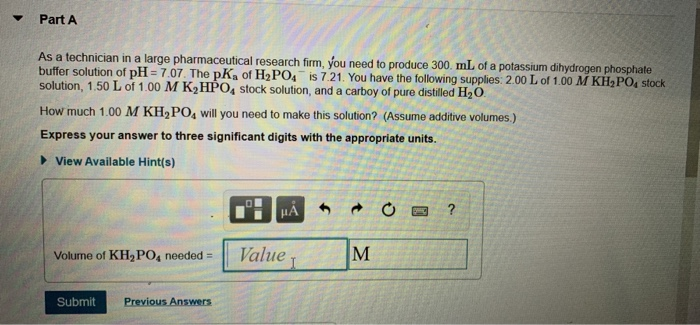Part A As a technician in a large pharmaceutical research firm, you need to produce 300 mL of a potassium dihydrogen phosphate buffer solution of pH = 7.07. The pK, of H2PO4 is 7.21. You have the following supplies: 2.00 L of 1.00 M KH2PO stock solution, 1.50 L of 1.00 M K HPO, stock solution, and a carboy of pure distilled H2O. How much 1.00 M KH PO, will you need to make this solution? (Assume additive volumes.) Express...

• ### As a technician in a large pharmaceutical research firm, you need to produce 400. mL of...

As a technician in a large pharmaceutical research firm, you need to produce 400. mL of a potassium dihydrogen phosphate buffer solution of pH = 6.93. The pKa of H2PO4− is 7.21. You have the following supplies: 2.00 L of 1.00 M KH2PO4 stock solution, 1.50 L of 1.00 M K2HPO4 stock solution, and a carboy of pure distilled H2O. How much 1.00 M KH2PO4 will you need to make this solution? (Assume additive volumes.) Express your answer to three...

• ### As a technician in a large pharmaceutical research firm, you need to produce 100. mL of...

As a technician in a large pharmaceutical research firm, you need to produce 100. mL of a potassium dihydrogen phosphate buffer solution of pH = 6.91. The p K a of H 2 P O 4 ? is 7.21. You have the following supplies: 2.00 L of 1.00 M KH2PO4 stock solution, 1.50 L of 1.00 M K2HPO4 stock solution, and a carboy of pure distilled H2O. How much 1.00 M KH2PO4 will you need to make this solution? (Assume...

• ### As a technician in a large pharmaceutical research firm, you need to produce 150.mL of 1.00...

As a technician in a large pharmaceutical research firm, you need to produce 150.mL of 1.00 M potassium dihydrogen phosphate buffer solution of pH = 6.79. The pKa of H2PO4? is 7.21. You have the following supplies: 2.00 L of 1.00 M KH2PO4 stock solution, 1.50 L of 1.00 M K2HPO4 stock solution, and a carboy of pure distilled H2O. How much 1.00 M KH2PO4 will you need to make this solution? Express your answer to three significant digits with...

• ### As a technician in a large pharmaceutical research firm, you need to produce 450.ml of 1.00M potassium phosphate buffer...

As a technician in a large pharmaceutical research firm, you need to produce 450.ml of 1.00M potassium phosphate buffer solution of pH = 7.13. The pKa of H2PO4 is 7.21. You have the following supplies: 2.00l of 1.00 KH2PO4 stock solution, 1.50 L of 1.00M KH2PO4 stock solution, and a carboy of pure distilled H2O How much 1.00 M KH2PO4 will you need to make this solution?

• ### 1) Calculate the pH of the solution that results from each of the following mixtures. A) 150.0 mL...

1) Calculate the pH of the solution that results from each of the following mixtures. A) 150.0 mL of 0.26 M  HF with 225.0 mL of 0.32 M  NaF B) 165.0 mL of 0.12 M C2H5NH2 with 275.0 mL of 0.22 M C2H5NH3Cl 2) A) As a technician in a large pharmaceutical research firm, you need to produce 100. mLof a potassium dihydrogen phosphate buffer solution of pH = 6.91. The pKa of H2PO4− is 7.21. You have the following...

• ### The Henderson-Hasselbalch equation relates the pH of a buffer solution to the pKa of its conjugat...

The Henderson-Hasselbalch equation relates the pH of a buffer solution to the pKa of its conjugate acid and the ratio of the concentrations of the conjugate base and acid. The equation is important in laboratory work that makes use of buffered solutions, in industrial processes where pH needs to be controlled, and in medicine, where understanding the Henderson-Hasselbalch equation is critical for the control of blood pH. Part A As a technician in a large pharmaceutical research firm, you need...

• ### Buffers

As a technician in a large pharmaceutical research firm, you need to produce 450 mL. of 1.00 M potassium phosphate buffer solution of pH = 7.36. The pKa of H2PO4-is 7.21.You have the following supplies: 2.00L of 1.00 M KH2PO4 stock solution, 1.50L of 1.00M K2HPO4 stock solution, and a carboy of pure distilled H2O. How much 1.00MKH2PO4 will you need to make this solution?

• ### Part A Calculate the pH of a 0.10 M solution of barium hydroxide, Ba(OH)2 Express your...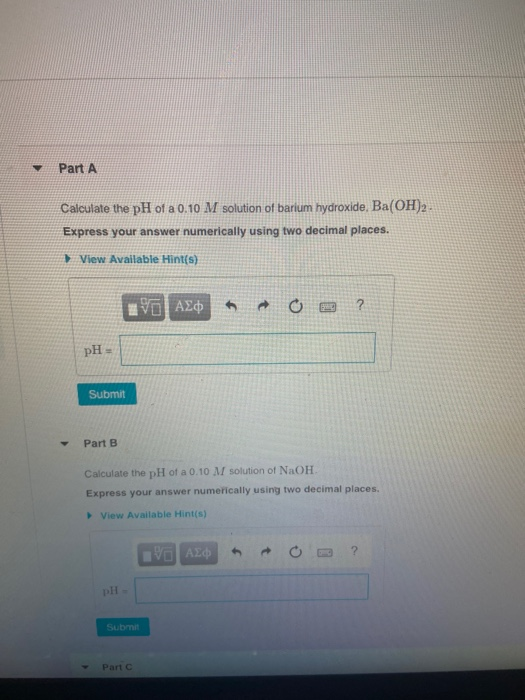Part A Calculate the pH of a 0.10 M solution of barium hydroxide, Ba(OH)2 Express your answer numerically using two decimal places. View Available Hint(s) 20 A20 0 2 ? pH Submit Part B Calculate the pH of a 0.10 M solution of NaOH Express your answer numerically using two decimal places. View Available Hint(s) VO ALDO ? Submit Part Part B ve a Calculate the pH of a 0.10 M solution of NaOH. Express your answer numerically using two...

• ### Part A You need to produce a buffer solution that has a pH of 5.14. You...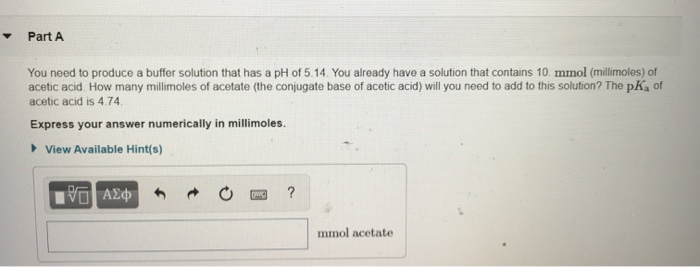Part A You need to produce a buffer solution that has a pH of 5.14. You already have a solution that contains 10. mmol (millimoles) of acetic acid. How many millimoles of acetate (the conjugate base of acetic acid) will you need to add to this solution? The pk of acetic acid is 4.74 Express your answer numerically in millimoles. View Available Hint(s) V AED ROO? mmol acetate Pall A beaker with 1 90x102 mL of an acetic acid buffer...

Free Homework App